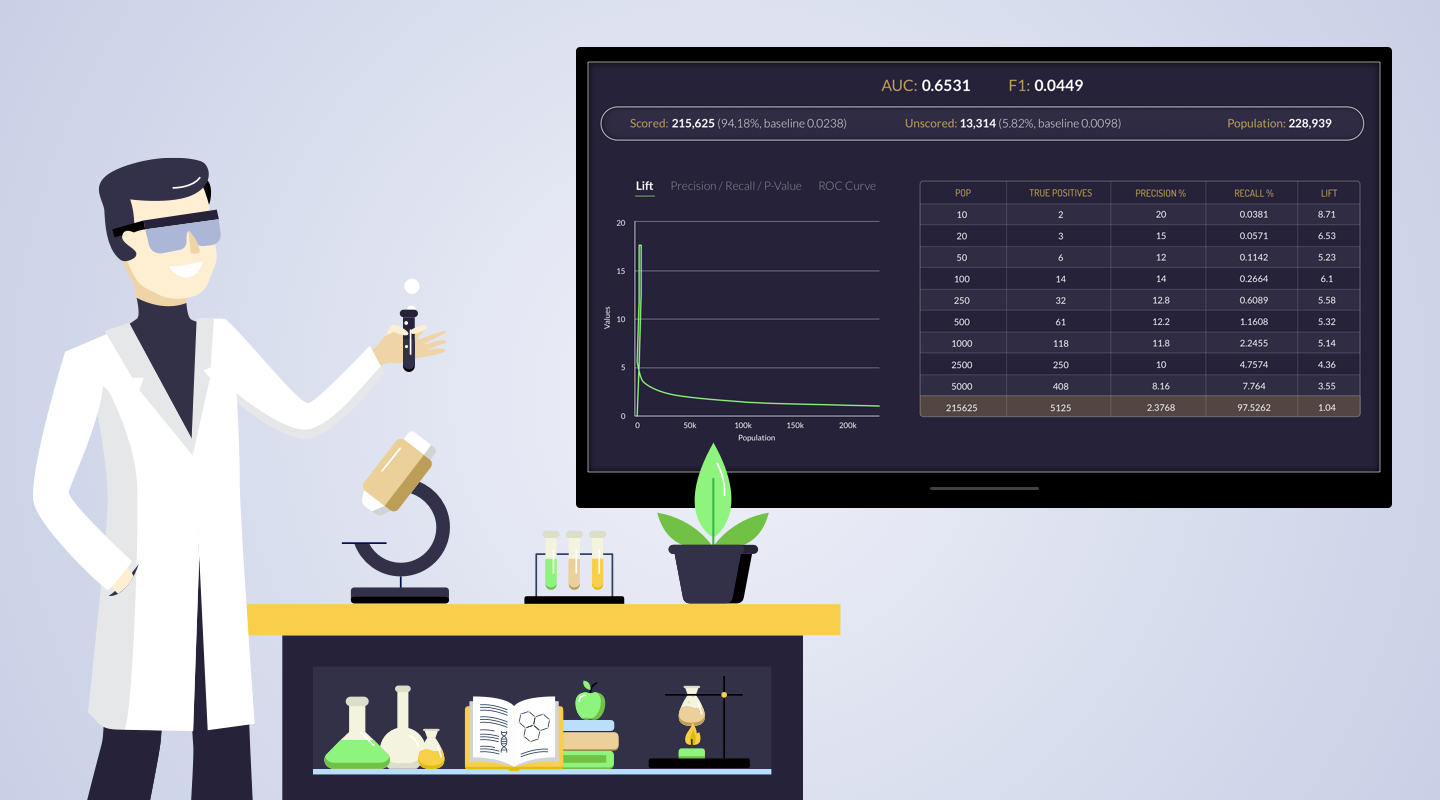Thank you!

Oops! Something went wrong while submitting the form.< USER GUIDE(protocol) How to evaluate the predictions using the top KPI measures available in the market?In order to have a better understanding as for the different KPIs of the prediction, the Past metrics screen, gives a wide coverage of all the KPIs in one screen.

Data and metrics are everywhere, and so are so called “Key Performance Indicators” (KPIs) - metrics that signal that a prediction is performing well (or not). Measuring and monitoring prediction performance is critical in order to have the confidence of acting upon it.

In order to have a better understanding as for the different KPIs of the prediction, the Past metrics screen, gives a wide coverage of all the KPIs in one screen. (The prediction is measured using a month of data which is hidden from it.) When the prediction runs, the system rolls 30 days back in time and runs the same prediction utilizing the examples and list to rank which is updaload in the prediction definition screen.

The available KPIs are:

AUC : Prediction accuracy is expressed as the correlation between the AMS prediction and the actual score. Accuracy of 1 indicates a perfect accuracy, whereas the accuracy of 0 indicates a random guess.

F1 Score : Balance between Precision and Recall.

Precision : Precision is a good measure to determine, when the costs of False Positive is high. For instance, email spam detection. In email spam detection, a false positive means that an email that is non-spam (actual negative) has been identified as spam (predicted spam). The email user might lose important emails if the precision is not high for the spam detection model.

Recall : actually calculates how many of the Actual Positives our model capture through labeling it as Positive (True Positive). Applying the same understanding, we know that Recall shall be the model metric we use to select our best model when there is a high cost associated with False Negative.

For instance, in fraud detection or sick patient detection. If a fraudulent transaction (Actual Positive) is predicted as non-fraudulent (Predicted Negative), the consequence can be very bad for the bank.

Similarly, in sick patient detection. If a sick patient (Actual Positive) goes through the test and predicted as not sick (Predicted Negative). The cost associated with False Negative will be extremely high if the sickness is contagious.

P-Value : Hypothetical frequency called the P-value, also known as the “observed significance level” for the test hypothesis. The traditional definition of P-value and statistical significance has revolved around null hypotheses, and we treat all other assumptions that are used to calculate P-value as if they are all correct. As we are not sure about these assumptions, we will learn about a more general view of the P-value as a statistical summary of the compatibility between the observed data and what we would predict or expect to see if we knew the entire statistical model were correct.

ROC Curve :Curves plot the true positive rate (sensitivity) against the false positive rate (1-specificity) for the different possible cutpoints of a diagnostic test.

Lift : is a measure of the effectiveness of a predictive model calculated as the ratio between the results obtained with and without the predictive model.

Scored : The audience that got ranked.

Unscored: The audience which were not scored as there were no behavioral significant signals to rank them by.